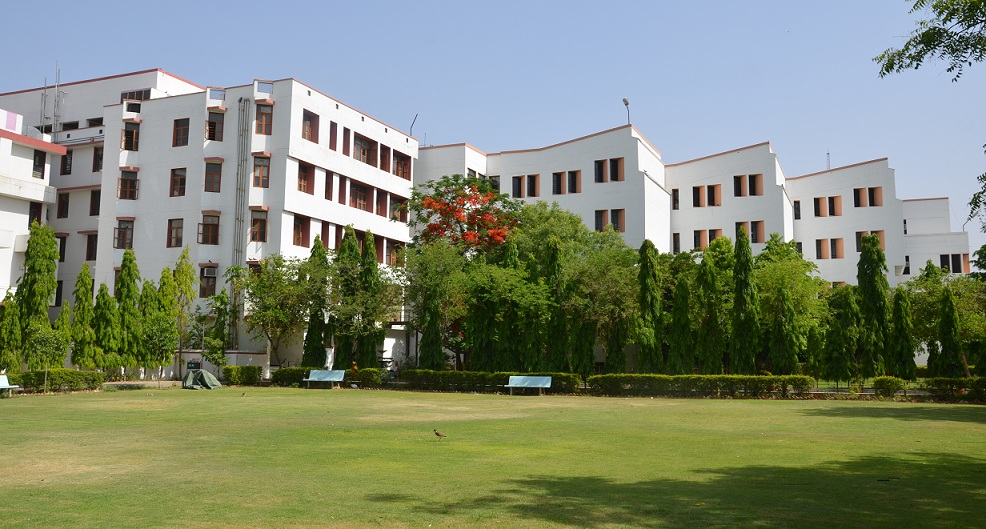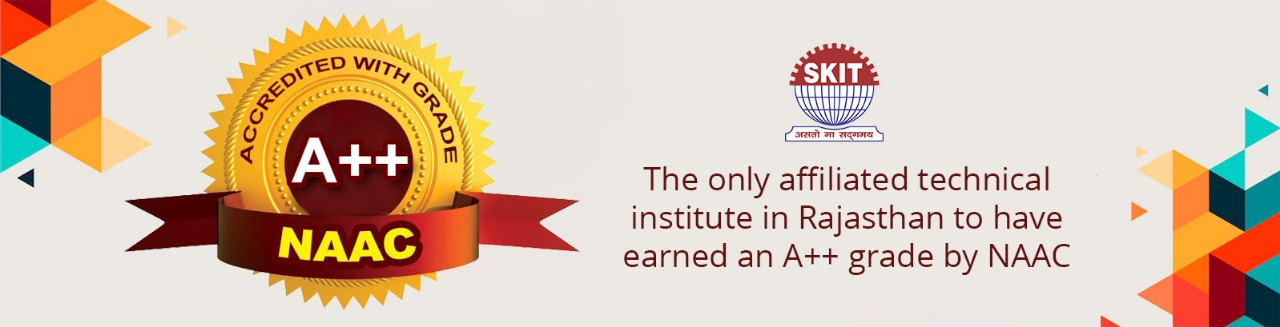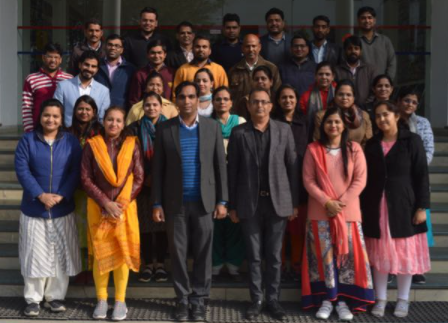﻿ ICASEMCT-2023
February 17-18, 2023
International Conference on “Advancements in Smart Electronics, Materials and Communication Technologies" (ICASEMCT-2023)
Jaipur, India

# // Set the date we're counting down to var countDownDate = new Date("Feb 17, 2023 09:00:00").getTime(); // Update the count down every 1 second var x = setInterval(function() { // Get todays date and time var now = new Date().getTime(); // Find the distance between now and the count down date var distance = countDownDate - now; // Time calculations for days, hours, minutes and seconds var days = Math.floor(distance / (1000 * 60 * 60 * 24)); var hours = Math.floor((distance % (1000 * 60 * 60 * 24)) / (1000 * 60 * 60)); var minutes = Math.floor((distance % (1000 * 60 * 60)) / (1000 * 60)); var seconds = Math.floor((distance % (1000 * 60)) / 1000); document.getElementById("demo").style.fontSize = "xx-large"; // Display the result in the element with id="demo" document.getElementById("demo").innerHTML = days + "d " + hours + "h " + minutes + "m " + seconds + "s "; // If the count down is finished, write some text if (distance < 0) { clearInterval(x); document.getElementById("demo").innerHTML = "EXPIRED"; } }, 1000);